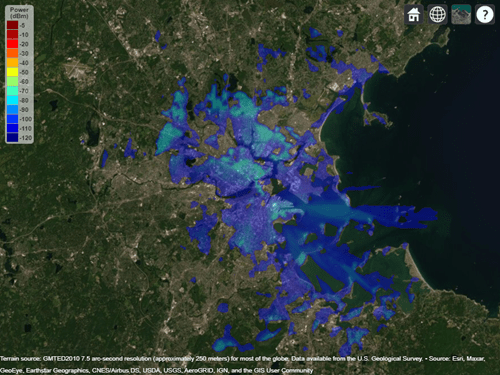# location

Coordinates of RF propagation data

Since R2020a

## Syntax

``datalocation = location(pd)``
``[lat,lon] = location(pd)``

## Description

````datalocation = location(pd)` returns the location coordinates of the data points in the propagation data object.```

example

````[lat,lon] = location(pd)` returns the latitude and longitude of the propagation data object```

## Examples

collapse all

Define names and locations of sites around Boston.

```names = ["Fenway Park","Faneuil Hall","Bunker Hill Monument"]; lats = [42.3467,42.3598,42.3763]; lons = [-71.0972,-71.0545,-71.0611];```

Create array of transmitter sites.

```txs = txsite("Name", names,... "Latitude",lats,... "Longitude",lons, ... "TransmitterFrequency",2.5e9);```

Compute received power data for each transmitter site.

```maxr = 20000; pd1 = coverage(txs(1),"MaxRange",maxr); pd2 = coverage(txs(2),"MaxRange",maxr); pd3 = coverage(txs(3),"MaxRange",maxr);```

Compute rectangle containing locations of all data.

```locs = [location(pd1); location(pd2); location(pd3)]; [minlatlon, maxlatlon] = bounds(locs);```

Create grid of locations over rectangle.

```gridlength = 300; latv = linspace(minlatlon(1),maxlatlon(1),gridlength); lonv = linspace(minlatlon(2),maxlatlon(2),gridlength); [lons,lats] = meshgrid(lonv,latv); lats = lats(:); lons = lons(:);```

Get data for each transmitter at grid locations using interpolation.

```v1 = interp(pd1,lats,lons); v2 = interp(pd2,lats,lons); v3 = interp(pd3,lats,lons);```

Create propagation data containing minimum received power values.

```minReceivedPower = min([v1 v2 v3],[],2,"includenan"); pd = propagationData(lats,lons,"MinReceivedPower",minReceivedPower);```

Plot minimum received power, which shows the weakest signal received from any transmitter site. The area shown may correspond to the service area of triangulation using the three transmitter sites.

```sensitivity = -110; contour(pd,"Levels",sensitivity:-5,"Type","power")```## Input Arguments

collapse all

Propagation data, specified as a `propagationData` object.

## Output Arguments

collapse all

Location of antenna site, returned as an M-by-`2` matrix with each element unit in degrees. M is the number of rows in the data table with valid latitude and longitude values. Duplicate locations are not removed.

Latitude of data points, returned as an M-by-`1` vector with each element unit in degrees.

Longitude of data points, returned as an M-by-`1` matrix with each element unit in degrees. The output is wrapped so that the values are in the range `[-180 180]`.

## Version History

Introduced in R2020a